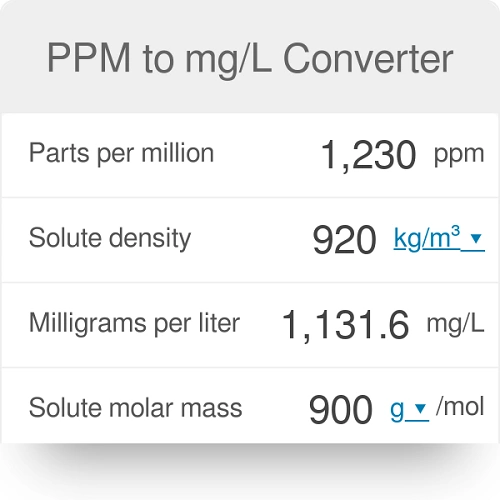# How To Convert From ppm To mg/lConcentrations of chemicals in water are typically measured in units of the mass of chemical (milligrams, mg or micrograms, ug) per volume of water (liter, L, l).

Concentrations in water can also be expressed as parts per million (ppm) or parts per billion (ppb). Parts per million and parts per billion may be converted from one to the other using this relationship: 1 part per million = 1,000 parts per billion.

For water, 1 ppm = approximately 1 mg/L (also written as mg/l) of contaminant in water, and 1 ppb = 1 ug/L (also written as ug/l). A measurement of 6 mg/L is the same as 6 ppm or 6,000 ppb, which is equal to 6,000 ug/L.

Read AlsoOccupational exposure: Examples and OEL

Occasionally, concentrations of chemicals in water may be written as grams per cubic meter (g/m3). This is the same as grams per 1,000 liters, which may be converted to milligrams per liter (mg/L).

Therefore, 1 g/m3 = 1 mg/L = 1 ppm. Likewise, one milligram per cubic meter (mg/m3)is the same concentration in water as one per
liter (ug/L), which is about 1 ppb.

Read AlsoHow to convert ppm to mg/m3 (Use NIOSH Calculator)